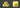Johann Pardanaud

# Mapping objects in JavaScriptJavaScript has been evolving fast these last years, but object mapping is still a tricky part for beginners, especially if you don't want to mutate the original object.

Here are some code examples for ES5, ES2015 and ES2017+. They all use the `map` and `reduce` methods available on arrays. These two methods allow to separate the mapping logic from the object construction.

For each example, the goal is to transform this object:

``````{
a: 1,
b: 2,
}``````

To this one:

``````{
_a: 2,
_b: 3,
}``````

### ES5

``````const data = {
a: 1,
b: 2,
}

const newData = Object.keys(data)
.map(function (key) {
return {
key: '_' + key,
value: data[key] + 1,
}
})
.reduce(function (obj, item) {
obj[item.key] = item.value
return obj
}, {})``````

### ES2015 (ES6)

``````const data = {
a: 1,
b: 2,
}

const newData = Object.keys(data)
.map((key) => ({ [`_\${key}`]: data[key] + 1 }))
.reduce((obj, item) => Object.assign(obj, item), {})``````

### ES2017+

``````const data = {
a: 1,
b: 2,
}

const newData = Object.entries(data)
.map(([key, value]) => ({ [`_\${key}`]: value + 1 }))
.reduce((obj, item) => Object.assign(obj, item), {})``````

### In the future

A new stage-0 proposal has been presented to add `Object.fromEntries`, this could provide us a new way to write the last example:

``````const data = {
a: 1,
b: 2,
}

const newData = Object.fromEntries(
Object.entries(data).map(([key, value]) => ({ [`_\${key}`]: value + 1 })),
)``````

And it would be even better with the pipeline operator (stage-1) to keep the method chaining:

``````const data = {
a: 1,
b: 2,
}

const newData =
Object.entries(data).map(([key, value]) => ({ [`_\${key}`]: value + 1 }))
|> Object.fromEntries``````